# Gestor de ecuaciones

### ZW3D 2017 Equation Manager Speeds up the Parametric Modeling Process

At the beginning of product design, the size and the shape of the model are usually uncertain, which need to be modified after various analysis and manufacture programming. Therefore, the model is supposed to be easy to modify. The parametric design nicely meets this requirement.

The parametric design is a design method based on dimension-driven feature modeling, which uses variables and equations to drive size of the model and control the shape. So variables and equations play important roles in parametric design.

The upgraded equation manager in ZW3D 2017 (Figure 1), with a lot of improved functions, helps users quickly create, manage and call variables. In addition, variables can also be imported from an Excel file and exported to an Excel table, being convenient to exchange data with ZW3D on other computers.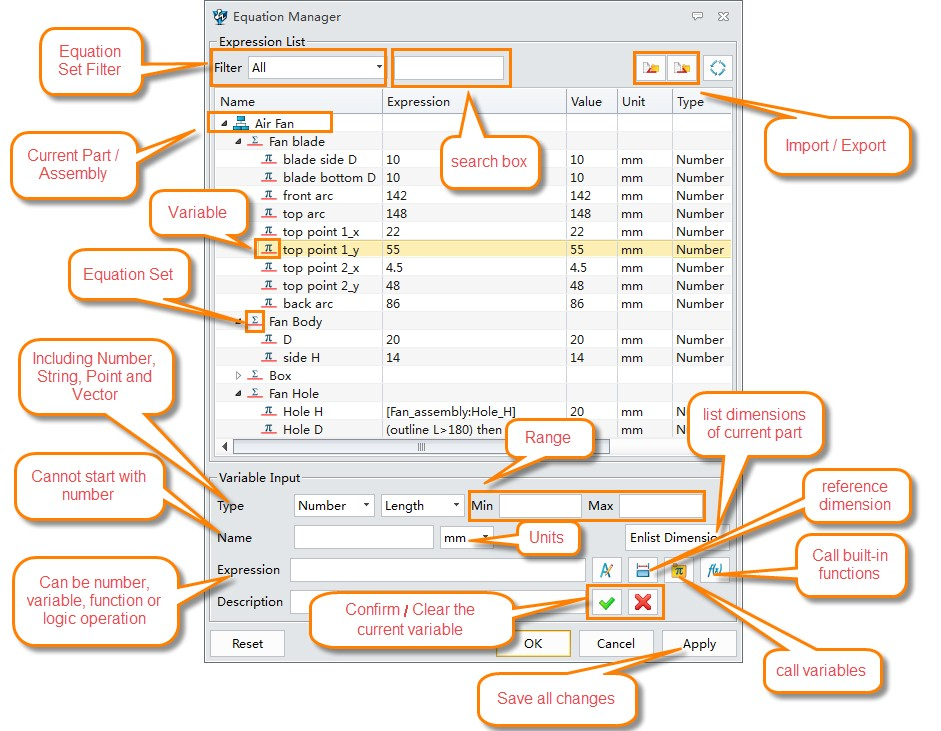Figure 1. New Equation Manager Form

How the equation manager performs an important role in the parametric modeling?

Next, we will introduce the related key points, including the creation of variable and equations, excel application, and parametric modeling.

1. Create Variables

Method 1: Directly create variables

In simple cases, users can directly create variables in the equation manager (Figure 2).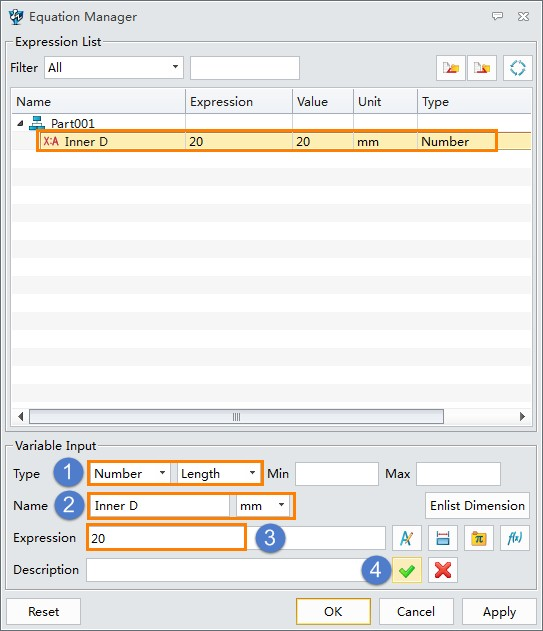Figure 2. Directly Create the Variable

Method2: Create variables in equation set

In ZW3D 2017, there is a newly-added equation set function in Equation Manger, which is used to group variables. Right click the blank area of list, then create a new equation set. For the complex product design, users can create multiple equation sets to manage variables according to different features of the model (Figure 3).Figure 3. Create Equation Set

Double click to activate one equation set, then create the variables in this equation set (Figure 4).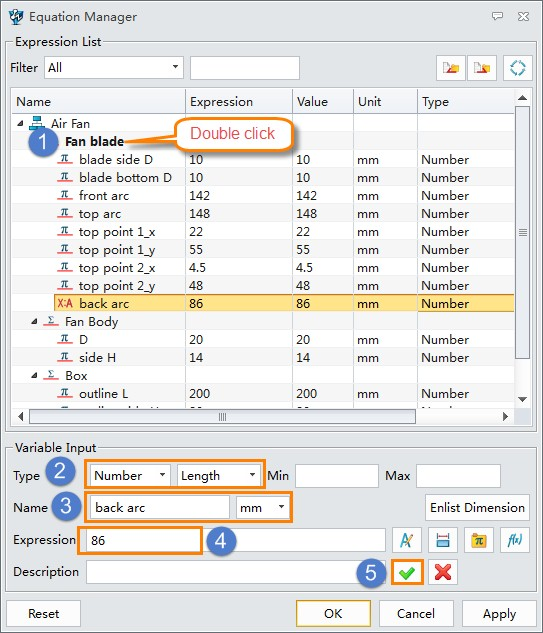Figure 4. Create Variable in Equation Set

2. Multi-import/export of Variables

Users can also create variables in an Excel file, then import them into ZW3D. In this way they can greatly increase efficiency of defining variables and shorten the period of parametric design (Figure 5).Figure 5. Import/Export of Variables

3. Call Variables

The defined variables can be called by the variable browser at any time.

1)  Call variables in sketch

After drawing the sketch outline, the constraints and dimensions should be added. During dimension definition or modification, the variables can be called to define the dimension value, as shown in figure 6. All variables are listed in the variable browser.

The parametric sketch, which should be well-defined to avoid failed update, changes with variables. This is the basis of the parametric modeling.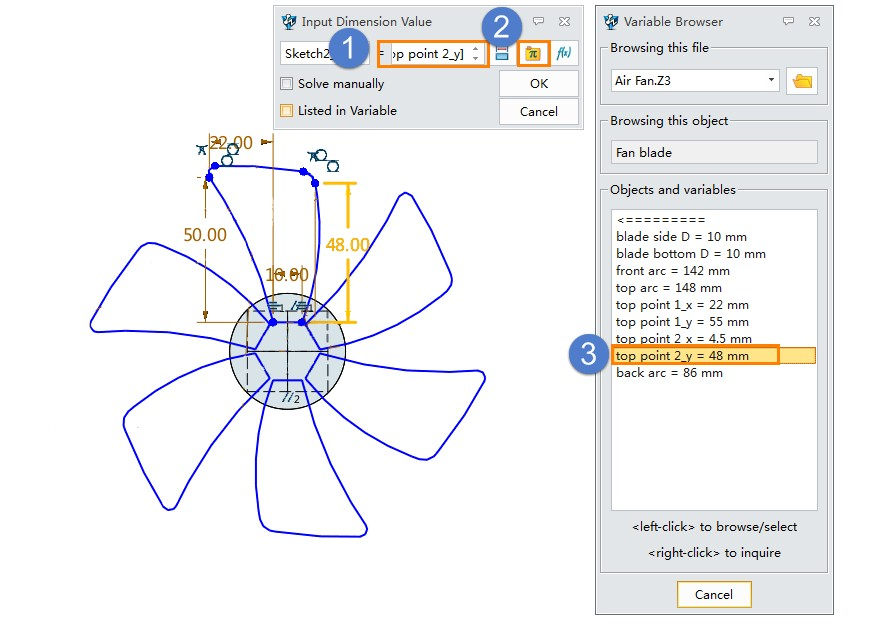Figure 6. Call Variables in Sketch

2)  Call variables in a single-part environment

During modeling, the variable can be used in any feature to define the model size and position. In the value input, the expression can be selectedfrom the right menu. Then we can use the variables or expression to define the feature values, see figure 7.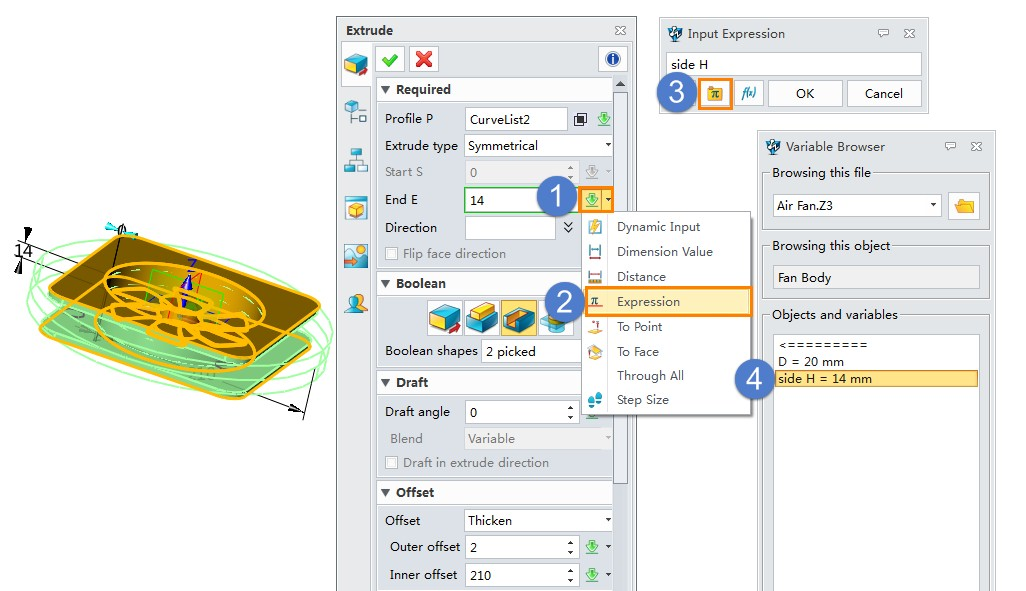Figure 7.Call Variables in Single-part Environment

3)  Call variables from external part

ZW3D 2017 supports calling variables from not only the current part but also external. In the variable browser, an option allows to browse variables from external part, as shown in figure 6.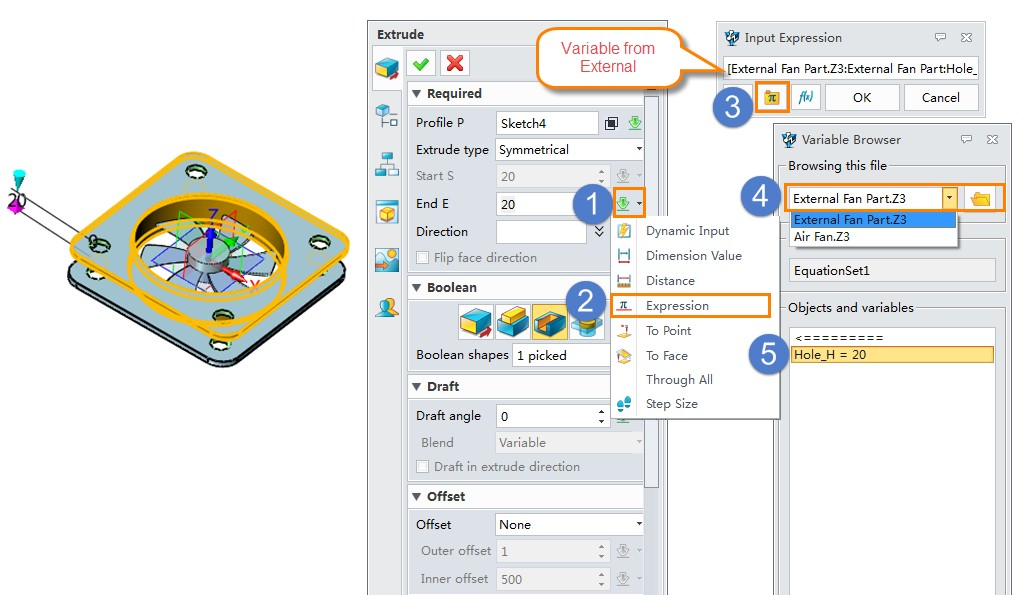Figure 8. Call Variables from External Part

4) Check back variables in equation manager

Variables called in sketch or from external will be listed in different variable types in the equation manager according to their sources. ZW3D 2017 provides a new filter to filtrate different types of variables so that users can quickly check back the infiltrated variables (Figure 9).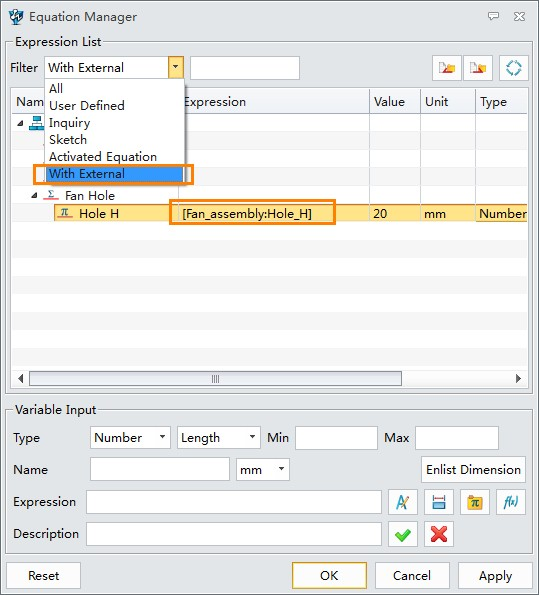Figure 9. The Filter Helps Inquire Variables

4. Verify the availability of the parameterized modeling

Variables created in the equation manager will be shown in history manage. Double click to quickly edit the variable. After modification, user should manually regenerate the model to finish the update and check the availability of the parameterized modeling (Figure 10).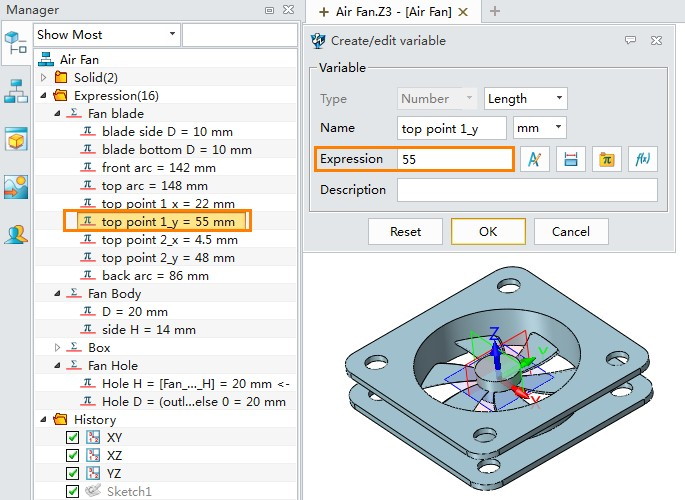Figure 10. Quick-edit the Variable

Based on a parametric model, in ZW3D users can customize a reused part library, which can be inserted as a component at any time (Figure 11).Figure 11. Reused Part Library

This article shows you how to use ZW3D 2017 new equation manager in parametric design. General process of parameterized modeling has been introduced. In ZW3D 2017 users can quickly define variables, call them at any time and at anywhere, manage them with groups,  and fast-edit them in history manager.

Please try ZW3D 2017 to experience more new features, such as dynamic section view and new sheet metal tools.